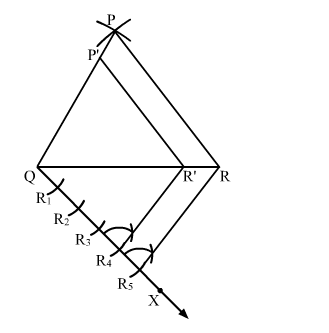# Construct a ∆PQR, in which PQ = 6 cm, QR = 7 cm and PR = 8 cm.`
Question:

Construct a $\triangle \mathrm{PQR}$, in which $\mathrm{PQ}=6 \mathrm{~cm}, \mathrm{QR}=7 \mathrm{~cm}$ and $\mathrm{PR}=8 \mathrm{~cm}$. Then, construct another triangle whose sides are $\frac{4}{5}$ times the corresponding sides of $\triangle \mathrm{PQR}$.

Solution:

Steps of Construction

Step 1. Draw a line segment QR = 7 cm.

Step 2. With Q as centre and radius 6 cm, draw an arc.

Step 3. With R as centre and radius 8 cm, draw an arc cutting the previous arc at P.

Step 4. Join PQ and PR. Thus, ∆PQR is the required triangle.

Step 5 . Below $Q R$, draw an acute angle $\angle R Q X$.

Step 6. Along QX, mark five points R1, R2, R3, Rand Rsuch that QR1 = R1R2 = R2R3 = R3R= R4R5.

Step 7. Join RR5.

Step 8. From R4, draw R4R' || RR5 meeting QR at R'.

Step 9. From R', draw P'R' || PR meeting PQ in P'.Here, $\triangle \mathrm{P}^{\prime} \mathrm{QR}^{\prime}$ is the required triangle, each of whose sides are $\frac{4}{5}$ times the corresponding sides of $\triangle \mathrm{PQR}$.